In :
import gzip
import json
from collections import Counter
from scipy.misc import factorial

In :
def Poisson(m,z=1): return np.exp(-z)*z**m/factorial(m)

In :
#list of events from 24 Sep '13
#each event has a key 'utc' and a key 'ip_hash'

In :
#list of events from 22 Sep '13

In :
#http://docs.python.org/2/library/time.html
from time import time,mktime,strptime,strftime,localtime

def ts2utc(ts):    #convert timestring to utc
return int(mktime(strptime(ts,'%d/%b/%Y:%H:%M:%S %Z')))

def utc2ts(utc):   #convert utc to timestring
return strftime('%d/%b/%Y:%H:%M:%S %Z',localtime(utc))

In :
for ts in '24/Sep/2013:10','24/Sep/2013:00','22/Sep/2013:00':
ts += ':00:00 EDT'
print ts,'\t',ts2utc(ts)

24/Sep/2013:10:00:00 EDT 	1380031200
24/Sep/2013:00:00:00 EDT 	1379995200
22/Sep/2013:00:00:00 EDT 	1379822400

In :
t= int(time())
days=t/86400
years=int(days/365.25)

print t,'seconds ago =',days,'days ago =',years,'years ago'
print 'so roughly',2016-years

1457638489 seconds ago = 16870 days ago = 46 years ago
so roughly 1970

In :
print t,'\t',utc2ts(t)
print '        ',0,'\t',utc2ts(0)

1457638489 	10/Mar/2016:14:34:49 EST
0 	31/Dec/1969:19:00:00 EST

In :
from urllib2 import urlopen
from IPython.display import Image

Out: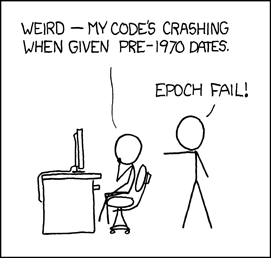In :
#create simple lists of utc's for all events in given timeframes
#use one hour periods to avoid averaging periods with too different average rates
# 24 Sep 10:00-11:00
t10= [e['utc'] for e in events if 1380031200 <= e['utc'] < 1380031200 + 3600 ]
# 24 Sep 00:00-01:00
tm = [e['utc'] for e in events if 1379995200 <= e['utc'] < 1379995200 + 3600 ]
# 22 Sep 00:00-01:00
tmw=[e['utc'] for e in wevents if 1379822400 <= e['utc'] < 1379822400 + 3600 ]

In :
#24 Sep 10:00-11:00, 24 Sep 00:00-01:00, 22 Sep 00:00-01:00
print len(t10),len(tm),len(tmw)
print 'averages:',map(lambda x: round(len(x)/3600.,3),[t10,tm,tmw]),'per second'

35315 23831 12176
averages: [9.81, 6.62, 3.382] per second

In :
import matplotlib.pyplot as plt
%matplotlib inline
import numpy as np

In :
scounts=Counter(tm).values()
zeroes = 3600-len(scounts)

plt.hist(scounts+*zeroes,np.arange(-.5,20),label='data')
plt.plot(3600*Poisson(np.arange(30),6.62),'r',lw=1.5,label='Poisson (avg=6.62/s)')
plt.xlabel('# web hits / second')
plt.ylabel('#seconds')
plt.title('arXiv.org web log data, 3600 seconds from 00:00-01:00 EDT 24 Sep 2013')
plt.xticks(range(0,31,2)), plt.xlim(-.5)
plt.legend();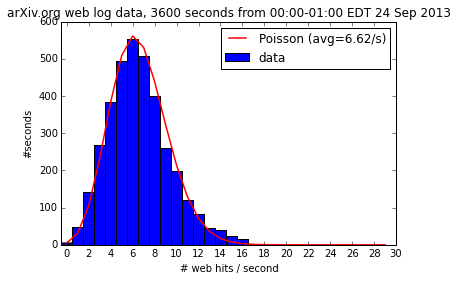In :
scounts=Counter(tmw).values()
zeroes = 3600-len(scounts)

plt.hist(scounts+*zeroes,np.arange(-.5,20),label='data')
plt.plot(3600*Poisson(np.arange(21),3.38),'r',lw=1.5,label='Poisson (avg=3.38/s)')
plt.xlabel('# web hits / second'), plt.ylabel('#seconds')
plt.title('arXiv.org web log data, 3600 seconds from 00:00-01:00 EDT 22 Sep 2013')
plt.xticks(range(21)), plt.xlim(-.5)
plt.legend();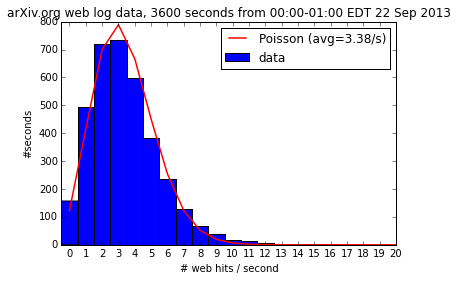In :
scounts=Counter(t10).values()
zeroes = 3600-len(scounts)

plt.hist(scounts+*zeroes,np.arange(-.5,30),label='data')
plt.plot(3600*Poisson(np.arange(30),9.81),'r',lw=1.5,label='Poisson (avg=9.81/s)')
plt.xlabel('# web hits / second')
plt.ylabel('#seconds')
plt.xticks(range(0,31,2)), plt.xlim(-.5)
plt.title('arXiv.org web log data, 3600 seconds from 10:00-11:00 EDT 24 Sep 2013')
plt.legend();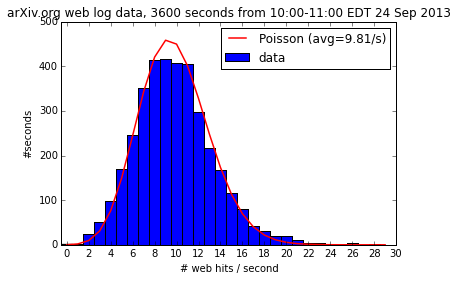This is not quite Poissonian, since a single host could be hitting multiple times (browser malfunction, multiple clicks, robotic access) and hence click times could be correlated. Instead can use distinct hosts/sec.

In :
mhosts={}
for e in events:
utc = e['utc']
if 1380031200 < utc < 1380031200+3600:  #24 Sep 2013 10:00
if utc not in mhosts: mhosts[utc]=[]
mhosts[utc].append(e['ip_hash'])

In :
#count distinct hosts / sec
dhostsm=map(lambda x:len(set(x)),mhosts.values())
zeroes = 3600-len(dhostsm)
np.mean(dhostsm)

Out:
9.2195664257921059
In :
plt.hist(dhostsm+*zeroes,np.arange(-.5,30),label='data')
p=Poisson(np.arange(24),9.22)
yerr=np.sqrt(3600*p*(1-p))
plt.errorbar(np.arange(24),3600*p,yerr,fmt='ro',lw=1.5,label='Poisson (avg=9.22/s)')
plt.xlabel('# distinct hosts / second')
plt.ylabel('#seconds')
plt.xticks(range(0,30,2)), plt.xlim(-.5)
plt.title('arXiv.org web log data, 3600 seconds from 10:00-11:00 EDT 24 Sep 2013')
plt.ylim(0,)
plt.legend();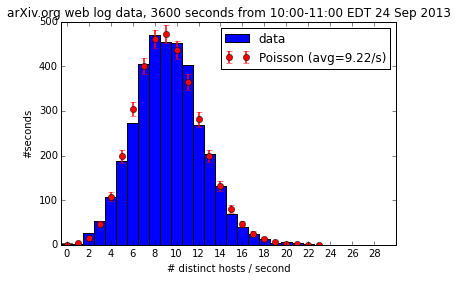If you want to play with any of this data, the following three files are available:
https://courses.cit.cornell.edu/info2950_2013fa/resources/130924_10.json.gz
https://courses.cit.cornell.edu/info2950_2013fa/resources/130924_00.json.gz
https://courses.cit.cornell.edu/info2950_2013fa/resources/130922_00.json.gz

They were created from utc's and ip_hashes for the three one-hour time periods as below:

In :
#create lists of utc's and ip_hashes for all events in given timeframes
# 24 Sep 10:00-11:00
e24Sep13_10= [{'utc':e['utc'],'ip_hash':e['ip_hash']}
for e in events if 1380031200 <= e['utc'] < 1380031200 + 3600 ]
# 24 Sep 00:00-01:00
e24Sep13_00= [{'utc':e['utc'],'ip_hash':e['ip_hash']}
for e in events if 1379995200 <= e['utc'] < 1379995200 + 3600 ]
# 22 Sep 00:00-01:00
e22Sep13_00= [{'utc':e['utc'],'ip_hash':e['ip_hash']}
for e in wevents if 1379822400 <= e['utc'] < 1379822400 + 3600 ]

json.dump(e24Sep13_10,gzip.open('130924_10.json.gz','w'))
json.dump(e24Sep13_00,gzip.open('130924_00.json.gz','w'))
json.dump(e22Sep13_00,gzip.open('130922_00.json.gz','w'))

In [ ]:


In :
#"galaxies" in the sky
n=10  #number of bins per axis
mu=3  #average number of points per bin
xdata,ydata=n*np.random.random([2,mu*n*n])

In :
plt.figure(figsize=(6.5,6))
plt.scatter(xdata,ydata);
plt.axis((0,10,0,10))
plt.grid('on');
plt.xticks(range(11))
plt.yticks(range(11));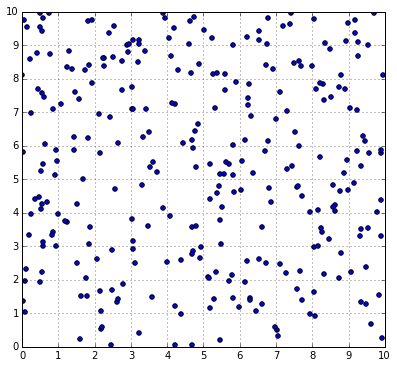In :
n=100
mu=3
data=n*np.random.random([mu*n*n,2])
integerbins= [(int(x),int(y)) for x,y in data]
counts=Counter(integerbins)
countvalues = [counts[(x,y)] for x in range(n) for y in range(n)]

plt.hist(countvalues,np.arange(-.5,3*mu))
p=Poisson(np.arange(15),mu)
yerr=np.sqrt(n*n*p*(1-p))
plt.errorbar(np.arange(15),n*n*p,yerr,fmt='ro')
plt.plot(np.arange(0,15,.1),n*n*Poisson(np.arange(0,15,.1),mu),'r-')
plt.ylim(0,)
plt.xlim(-.5,15);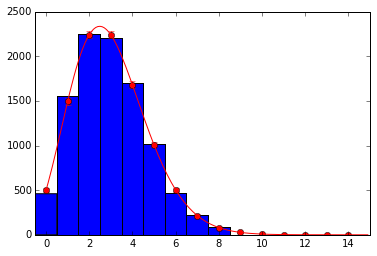The above closely matches a Poisson distribution.
In class I reran the above for values of $n$ equal to 10, 100, and 1000, to show how the number counts fluctuate much more from the Poission values for smaller values of $n$. The effective number of trials is $N=n^2$ since that is the number of squares in the 2d grid where data points are counted. Each number count can be considered a Bernoulli trial with probability of success given by the Poisson probability. For example, the probability of counting 2 data points in a bin is given by $q=p(2)\approx .22$, so the expected value is given by $Nq$, the variance by $Nq(1-q)$, and the relative fractional deviation by $\sqrt{Nq(1-q)} \big/ Nq = \sqrt{(1-q)/q}\Big/n$. This is roughly 2% for $q=.22$ and $n=100$, which is why the agreement for the bin counting 2's looks good. For $n=10$, it becomes 20%, so the numbers visibly fluctuate much more from run to run. A similar estimate can be made for each number count $m=0,1,\ldots$, using $q=p(m)$.

In [ ]: